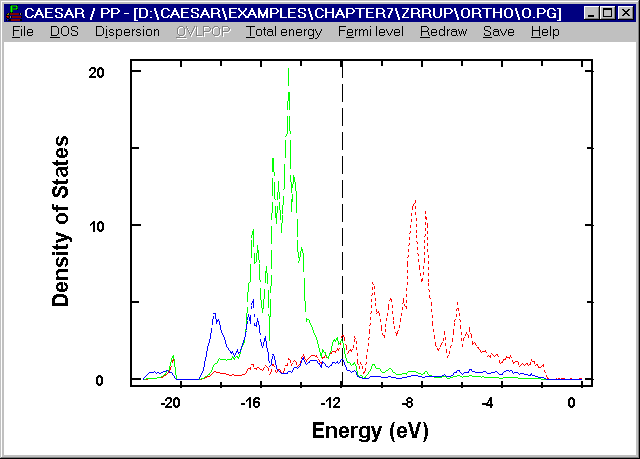# PDOS of o-ZrRuP

Two structural types are known for ZrRuP, i.e., the Fe2P-type hexagonal structure (h-ZrRuP) and the Co2P-type orthorhombic structure (o-ZrRuP). Superconductivity is found for both phases, but the superconducting transition temperature Tc is higher for h-ZrRuP than for o-ZrRuP (i.e., 13.0 versus 3.5 K).4 Specific heat measurements for ZrRuP show that the Debye temperature QD, which is a measure of the lattice stiffness, is lower for h-ZrRuP than for o-ZrRuP (345 versus 454 K).5 Specific heat measurements 6 also reveal that the number of electronic states at the Fermi level, i.e., n(ef), is low for h-ZrRuP, i.e., 1.52 electrons/(eV.atom).

The following figure shows the PDOS plots of the Zr, Ru and P atoms calculated for o-ZrRuP. In general, the PDOS plots of o-ZrRuP are similar to those of h-ZrRuP. However, the major contributions of Zr and Ru are spread over a wider region of energy in o-ZrRuP, which means stronger interactions between Zr and Ru in o-ZrRuP than in h-ZrRuP. This reflects the fact that the Zr-Ru distances are shorter in o-ZrRuP (2.903, 2 x 2.926, 2.967, 2 x 2.982 Å) than in h-ZrRuP (2 x 2.95, 4 x 3.00 Å). The Fermi level ef of o-ZrRuP occurs where the DOS is low. The n(ef) value of o-ZrRuP, 0.25 states/(eV.atom), is small but is slightly larger than that of h-ZrRuP.Figure. PDOS plots calculated for the Zr, Ru and P atoms of o- ZrRuP. The solid, dotted and dashed lines refer to the Zr, Ru and P atoms, respectively.

Go back to The Gallery